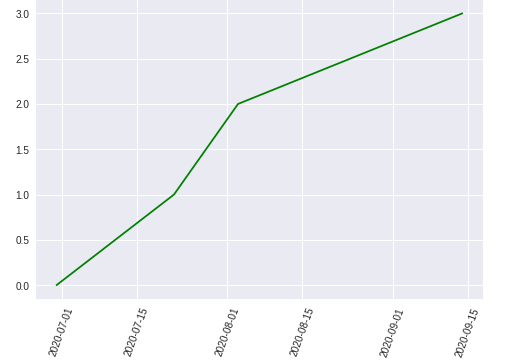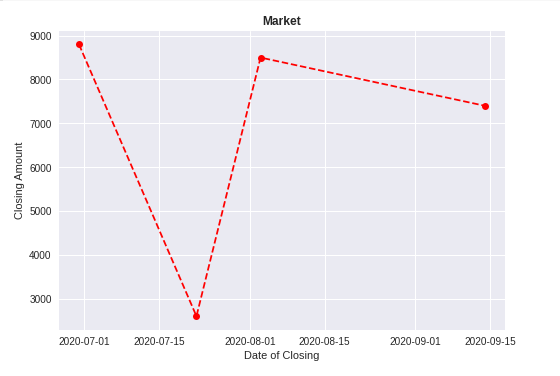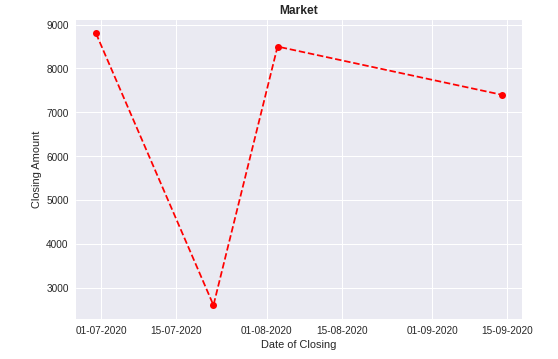# Matplotlib.pyplot.plot_date() function in Python

• Last Updated : 23 Dec, 2020

Matplotlib is a module or package or library in python which is used for data visualization. Pyplot is an interface to a Matplotlib module that provides a MATLAB-like interface.

## Matplotlib.pyplot.plot_date ()

This function  used to add dates to the plot.

Attention geek! Strengthen your foundations with the Python Programming Foundation Course and learn the basics.

To begin with, your interview preparations Enhance your Data Structures concepts with the Python DS Course. And to begin with your Machine Learning Journey, join the Machine Learning - Basic Level Course

Syntax:

matplotlib.pyplot.plot_date(x, y, fmt=’o’, tz=None, xdate=True, ydate=False,  data=None, **kwargs)

This is the syntax of date function. It contains various parameters or arguments which are explained below.

The last parameter **kwargs is the Keyword arguments control the Line2D properties like animation, dash_ joint-style, colors, linewidth, linestyle, marker, etc.

Example 1:

## Python3

 `# importing libraries``import` `matplotlib.pyplot as plt``from` `datetime ``import` `datetime`` ` `# creating array of dates for x axis``dates ``=` `[``    ``datetime(``2020``, ``6``, ``30``),``    ``datetime(``2020``, ``7``, ``22``),``    ``datetime(``2020``, ``8``, ``3``),``    ``datetime(``2020``, ``9``, ``14``)``]`` ` `# for y axis``x ``=` `[``0``, ``1``, ``2``, ``3``]`` ` `plt.plot_date(dates, x, ``'g'``)``plt.xticks(rotation``=``70``)``plt.show()`

Output:Example 2: Creating a plot using dataset.

## Python3

 `# importing libraries``import` `pandas as pd``import` `matplotlib.pyplot as plt``from` `datetime ``import` `datetime`` ` `# creating a dataframe``data ``=` `pd.DataFrame({``'Date'``: [datetime(``2020``, ``6``, ``30``),``                              ``datetime(``2020``, ``7``, ``22``),``                              ``datetime(``2020``, ``8``, ``3``),``                              ``datetime(``2020``, ``9``, ``14``)],``                      ` `                     ``'Close'``: [``8800``, ``2600``, ``8500``, ``7400``]})`` ` `# x-axis``price_date ``=` `data[``'Date'``]`` ` `# y-axis``price_close ``=` `data[``'Close'``]`` ` `plt.plot_date(price_date, price_close, linestyle``=``'--'``, color``=``'r'``)``plt.title(``'Market'``, fontweight``=``"bold"``)``plt.xlabel(``'Date of Closing'``)``plt.ylabel(``'Closing Amount'``)`` ` `plt.show()`

#### Output:Example 3: Changing the format of the date:

## Python3

 `# importing libraries``import` `pandas as pd``import` `matplotlib.pyplot as plt``from` `datetime ``import` `datetime`` ` `# creating a dataframe``data ``=` `pd.DataFrame({``'Date'``: [datetime(``2020``, ``6``, ``30``), ``                              ``datetime(``2020``, ``7``, ``22``), ``                              ``datetime(``2020``, ``8``, ``3``),``                              ``datetime(``2020``, ``9``, ``14``)],``                      ` `                     ``'Close'``: [``8800``, ``2600``, ``8500``, ``7400``]})`` ` `# x-axis``price_date ``=` `data[``'Date'``]`` ` `# y-axis``price_close ``=` `data[``'Close'``]`` ` `plt.plot_date(price_date, price_close, linestyle``=``'--'``, color``=``'r'``)``plt.title(``'Market'``, fontweight``=``"bold"``)``plt.xlabel(``'Date of Closing'``)``plt.ylabel(``'Closing Amount'``)`` ` `# Changing the formate of the date using``# dateformatter class``format_date ``=` `mpl_dates.DateFormatter(``'%d-%m-%Y'``)`` ` `# getting the accurate current axes using gca()``plt.gca().xaxis.set_major_formatter(format_date)`` ` `plt.show()`

Output:The format of the date changed to dd-mm-yyyy. To know more about dataformatter and gca() click here.

My Personal Notes arrow_drop_up